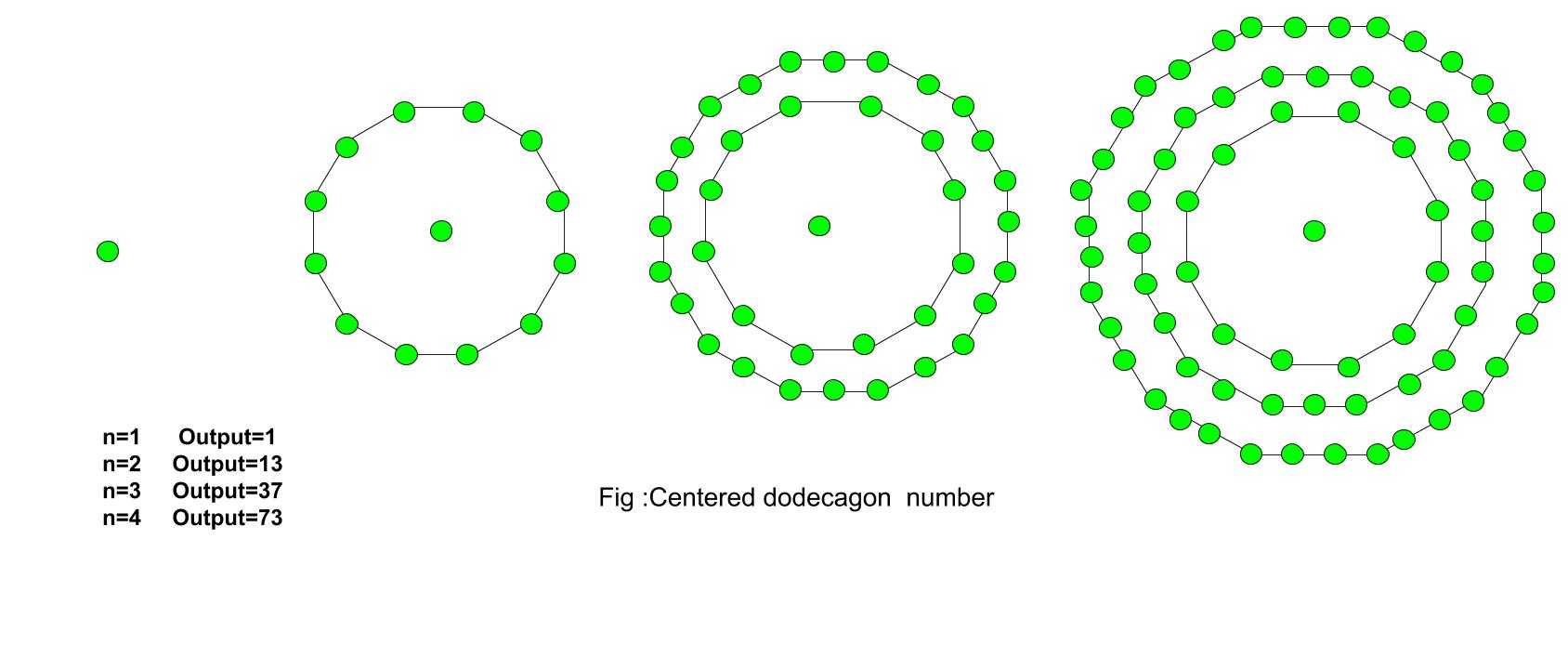# Centered Dodecagonal Number

Given a number n, find the nth Centered Dodecagonal Number.
The Centered Dodecagonal Number represents a dot in the center and other dots surrounding it in successive dodecagonal(12 sided polygon) layers.

Examples :

```Input :  3
Output : 37

Input : 7
Output :253
```The first few centered dodecagonal numbers are:
1, 13, 37, 73, 121, 181, 253, 337, 433, 541, 661…………………..

The formula for the nth Centered dodecagonal number:

``````

## C++

 `// C++ Program to find ` `// nth centered ` `// Dodecagonal number ` `#include ` `using` `namespace` `std; ` ` `  `// Function to calculate Centered ` `// Dodecagonal number ` `int` `centeredDodecagonal(``long` `int` `n) ` `{ ` `    ``// Formula to calculate nth ` `    ``// centered Dodecagonal number ` `    ``return` `6 * n * (n - 1) + 1; ` `} ` ` `  `// Drivers Code ` `int` `main() ` `{ ` `    ``long` `int` `n = 2; ` `    ``cout << centeredDodecagonal(n); ` `    ``cout << endl; ` `    ``n = 9; ` `    ``cout << centeredDodecagonal(n); ` ` `  `    ``return` `0; ` `} `

## Java

 `// Java Program to find ` `// nth centered ` `// Dodecagonal number ` `import` `java.io.*; ` ` `  `class` `GFG  ` `{ ` `// Function to calculate  ` `// Centered Dodecagonal number ` `static` `long` `centeredDodecagonal(``long` `n) ` `{ ` `    ``// Formula to calculate nth ` `    ``// centered Dodecagonal number ` `    ``return` `6` `* n * (n - ``1``) + ``1``; ` `} ` ` `  `// Driver Code ` `public` `static` `void` `main (String[] args)  ` `{ ` `    ``long` `n = ``2``; ` `    ``System.out.println(centeredDodecagonal(n)); ` ` `  `    ``n = ``9``; ` `    ``System.out.println(centeredDodecagonal(n)); ` `} ` `} ` ` `  `// This code is contributed by anuj_67. `

Output :

```13
433
```

References
http://oeis.org/A003154

My Personal Notes arrow_drop_upCheck out this Author's contributed articles.

If you like GeeksforGeeks and would like to contribute, you can also write an article using contribute.geeksforgeeks.org or mail your article to contribute@geeksforgeeks.org. See your article appearing on the GeeksforGeeks main page and help other Geeks.

Please Improve this article if you find anything incorrect by clicking on the "Improve Article" button below.

Improved By : vt_m